# 光栅化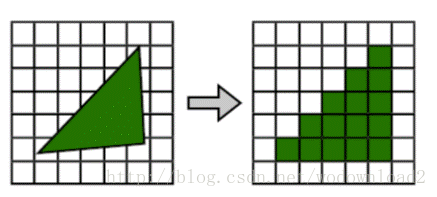# 重点部分及实现

## 采用obj模型，ppm图片贴图，双线性过滤进行采样

 1  usemtl chess.ppm

  1 2 3 4 5 6 7 8 9 10 11 12 13 14 15 16 17 18 19 20 21 22 23 24 25  RGB_T Texture::Sample(double u, double v) const { if(pixels.empty()) { return RGB_T(0.9); } // (-inf, +inf) u *= size; v *= size; // (-inf * size, +inf * size) auto f = [&](double a, double b) { int x = floor(u + a); int y = floor(v + b); x = ((x % size) + size) % size; y = ((y % size) + size) % size; return pixels[size * y + x] * fabs((u + a) - floor(u + 0.5)) * fabs((v + b) - floor(v + 0.5)); }; RGB_T ret = f(0.5, 0.5); ret += f(0.5, -0.5); ret += f(-0.5, 0.5); ret += f(-0.5, -0.5); return ret; } 

## 支持方向光和点光源两种光源

 1 2  virtual RGB_T Sample(const Vector4D_T &p, const Vector4D_T &n) = 0; 

### 方向光

 1 2 3 4 5 6  RGB_T DirectLight::Sample(const Vector4D_T &p, const Vector4D_T &n) { double NdotD = Dot(n, VD); return color * std::max(0.0, -NdotD); } 

### 点光源

 1 2 3 4 5 6 7 8  RGB_T PointLight::Sample(const Vector4D_T &p, const Vector4D_T &n) { double length2 = (p - VP).Length2(); Vector4D_T direction = Normalize(p - VP); double NdotD = Dot(n, direction); return color * std::max(0.0, -NdotD) / length2; } 

## Z-Buffer保证渲染正确的顺序，背面消影和三角形剔除(未实现三角形裁剪)

### Z-Buffer

Z-Buffer我是创建了一个double类型的vector分辨率和输出分辨率一样来存储z值即深度。在每次渲染新的像素到结果的时候都会判断一下当前z值是否比之前的小，小就代表在之前像素的前面，这样就可以正确的实现先后顺序。这里因为z值预先变成了1/z所以是>=。

 1 2 3 4 5  if(OnePreZ >= depth[y * width + x]) { ... } 

### 背面消隐

 1 2 3 4 5  bool Render::IsBackFace(const Vector4D_T &v0, const Vector4D_T &v1, const Vector4D_T &v2) { return Dot(v0, Cross(v1 - v0, v2 - v0)) >= 0; } 

### 三角形剔除

 1 2 3 4 5 6  if(v[i].point.z < 0.0 || v[i].point.z > 1.0) { OutOfRange = true; break; } 

# 渲染结果

## 不同贴图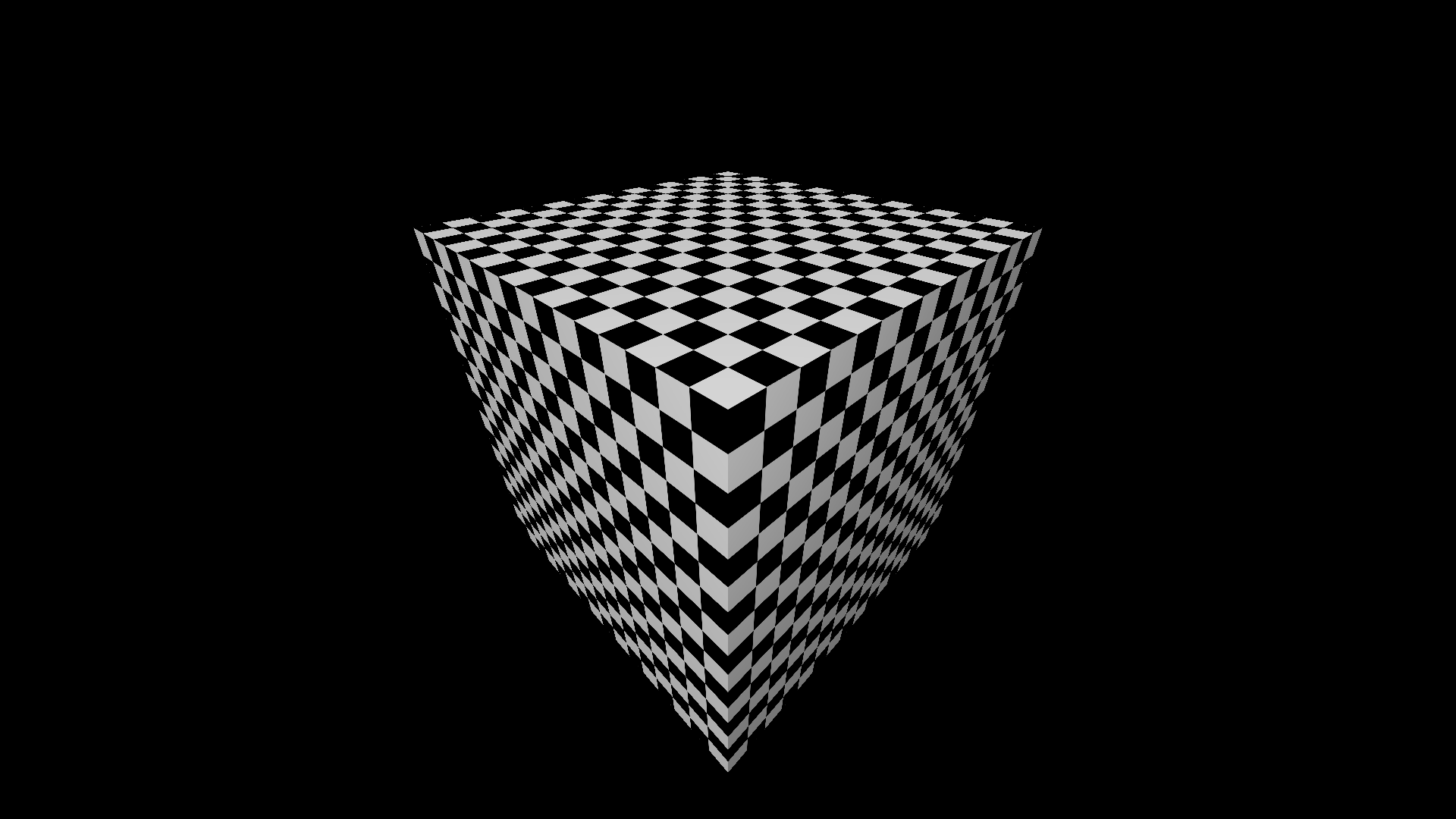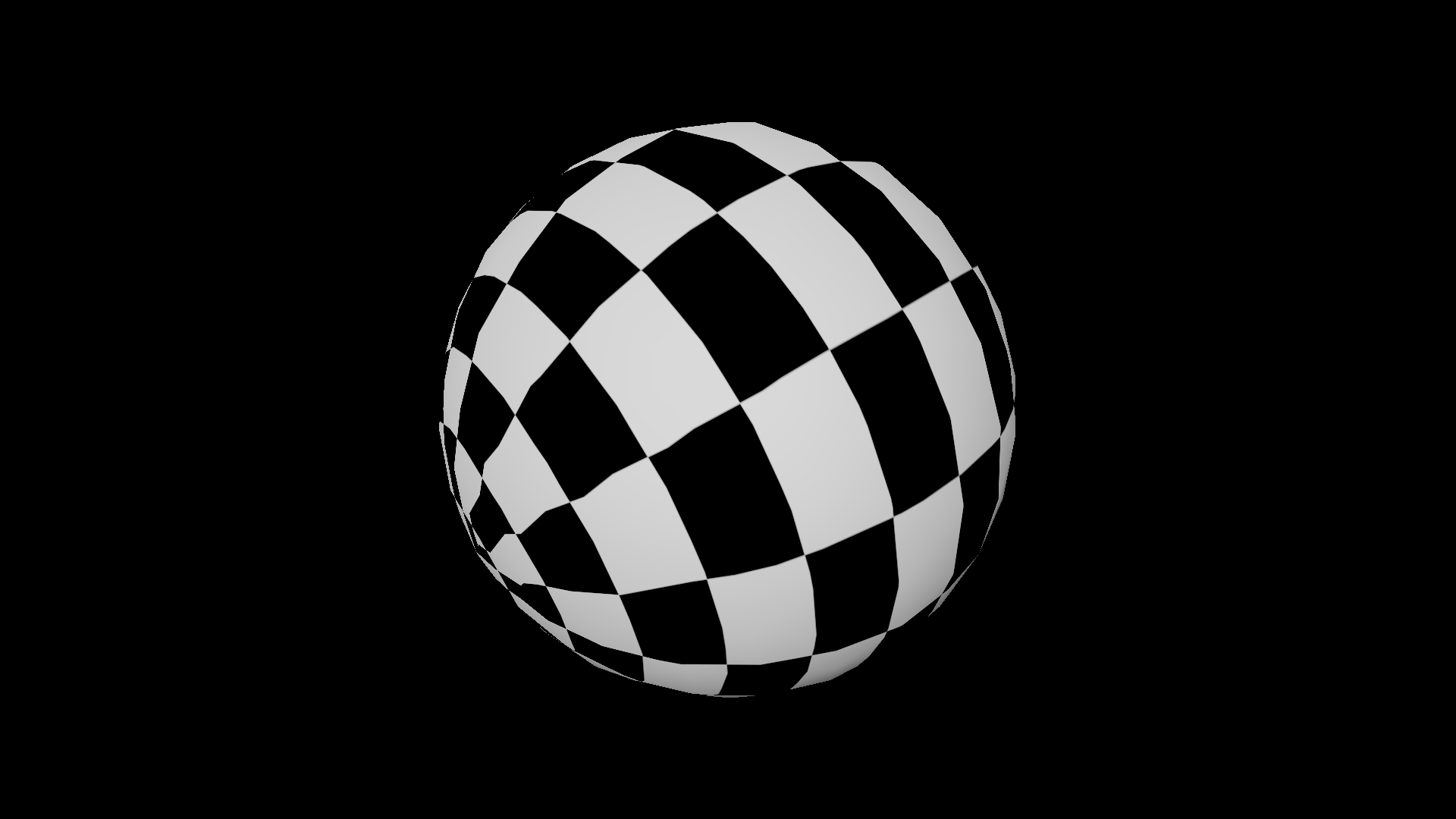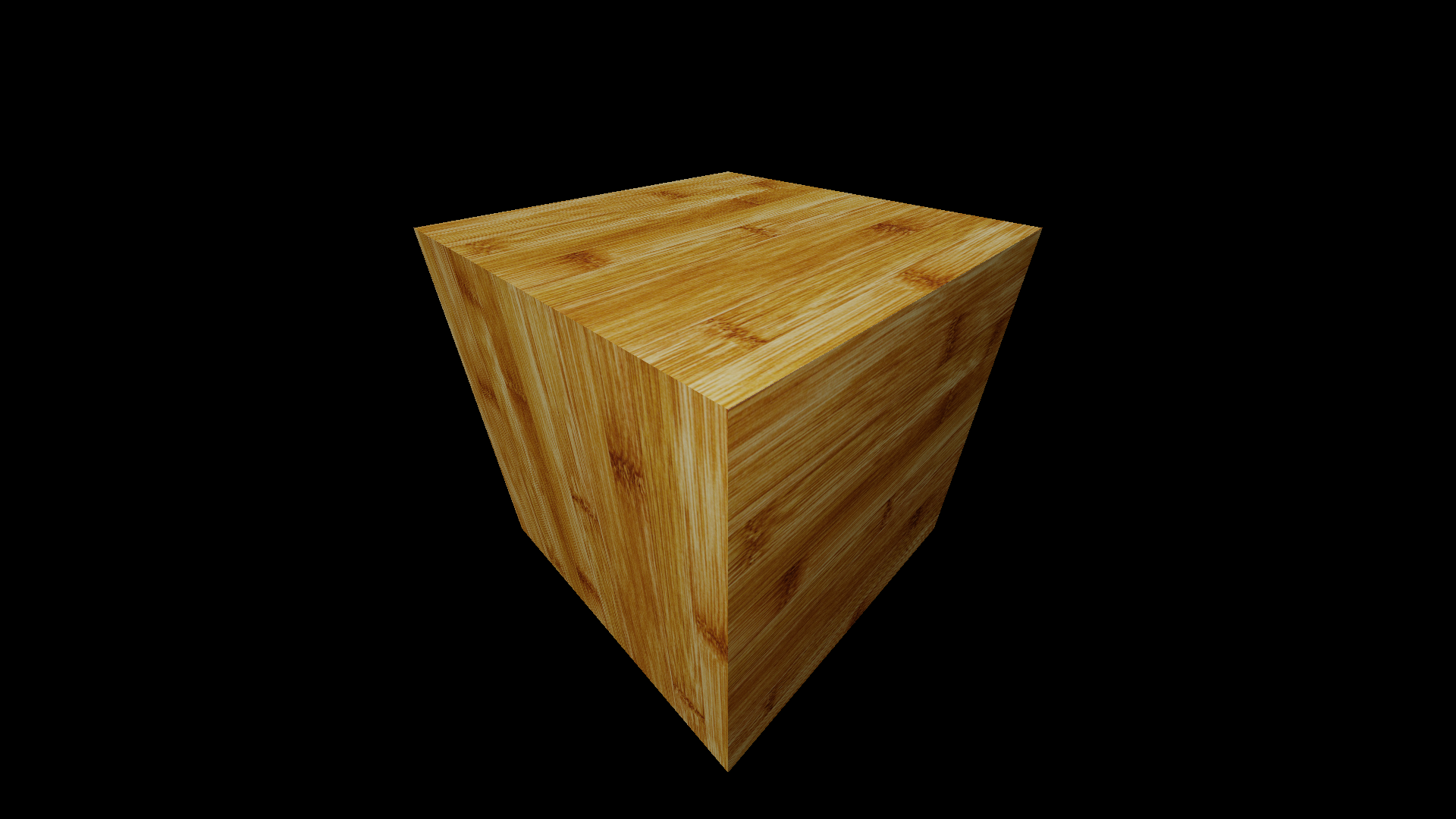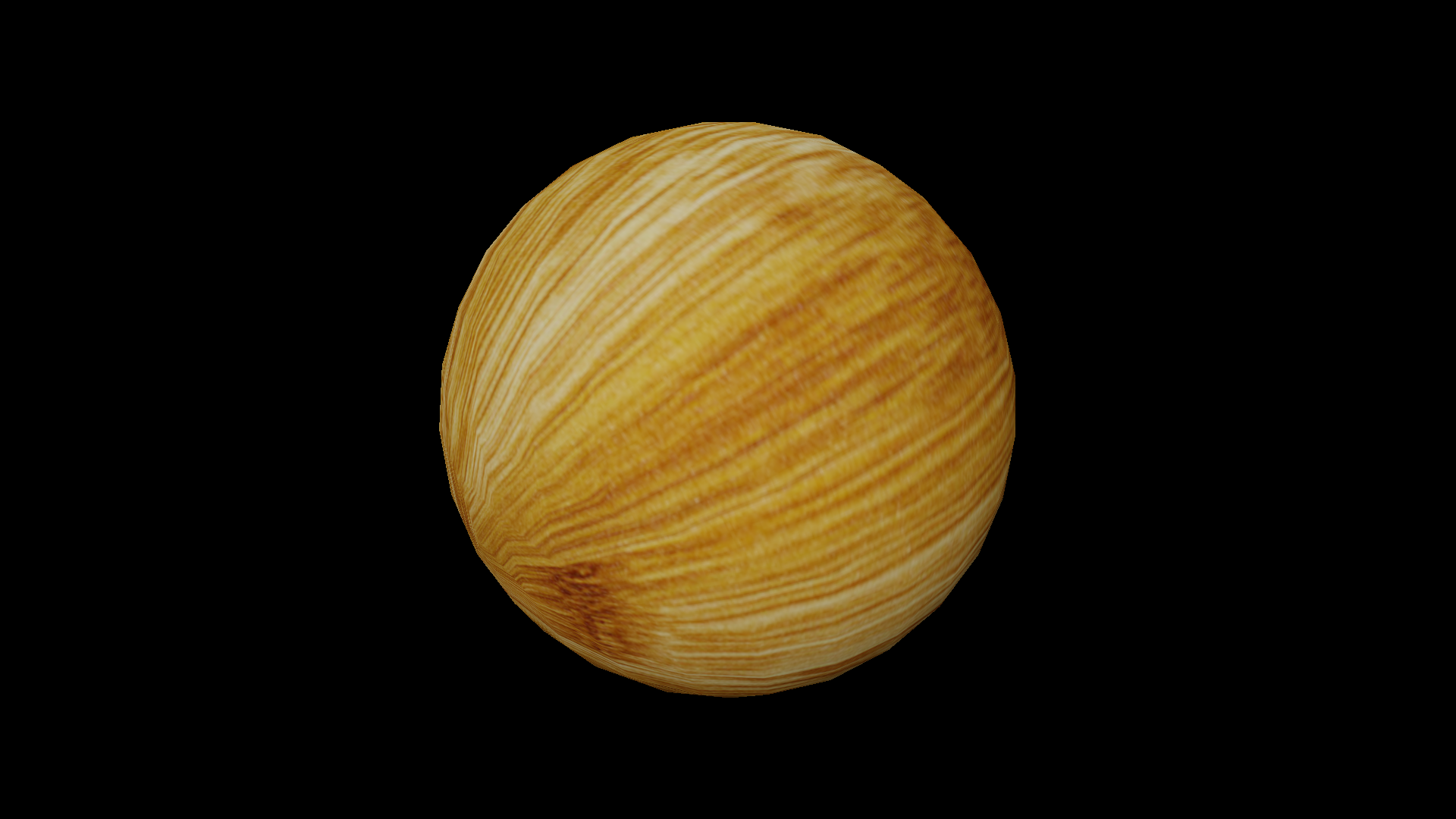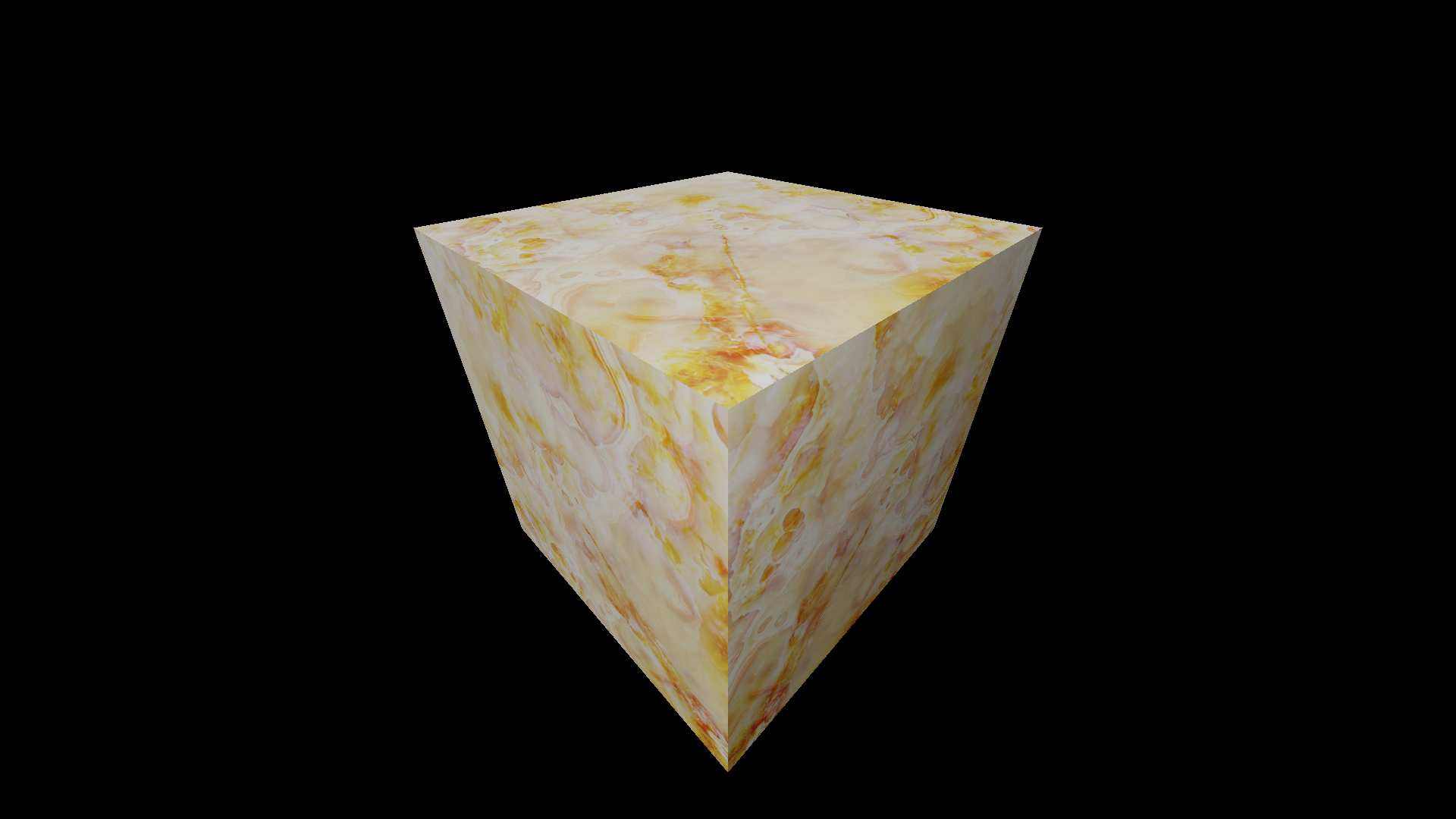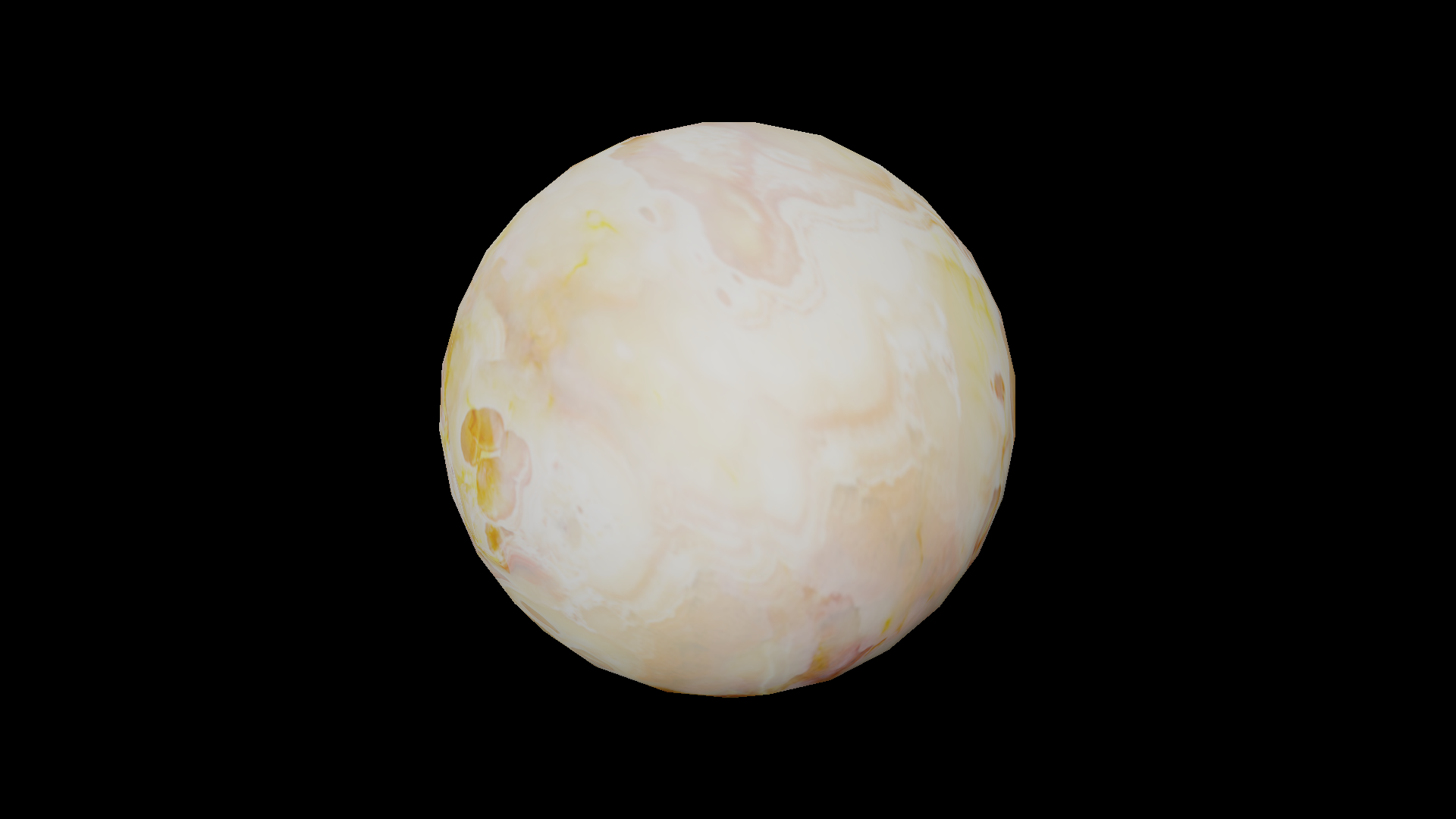## 斯坦福兔子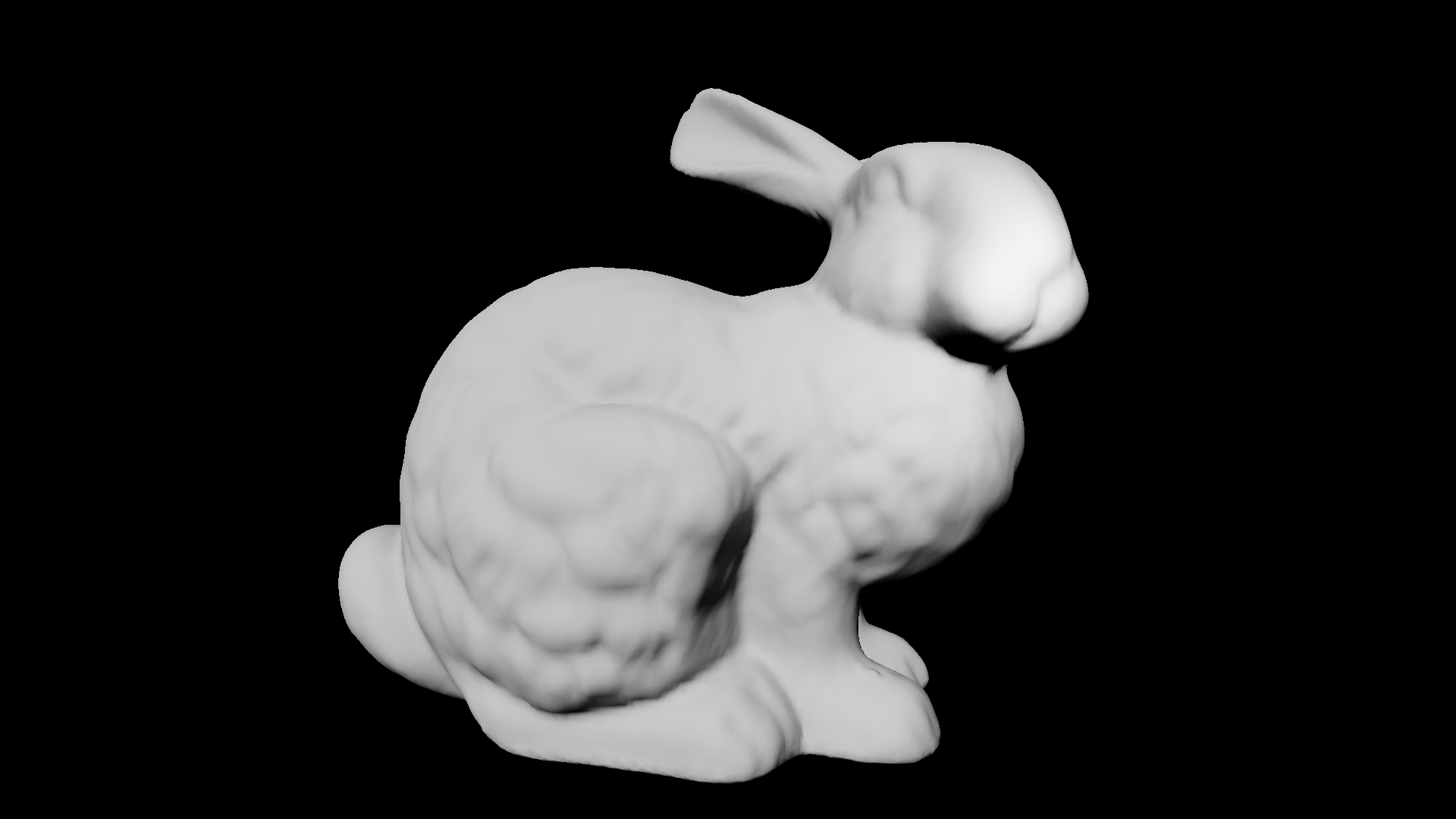## 不同光源 （平行光和点光源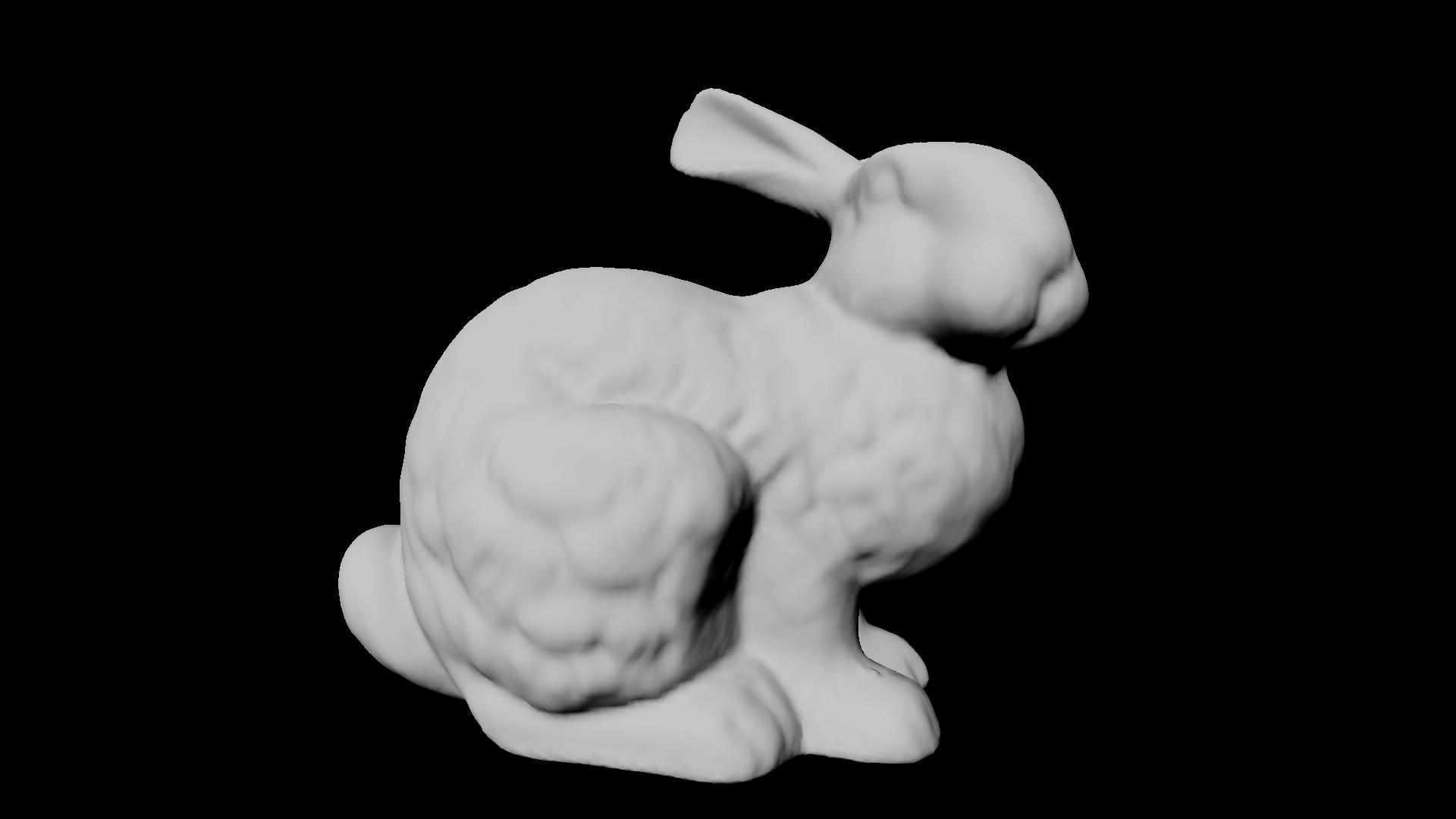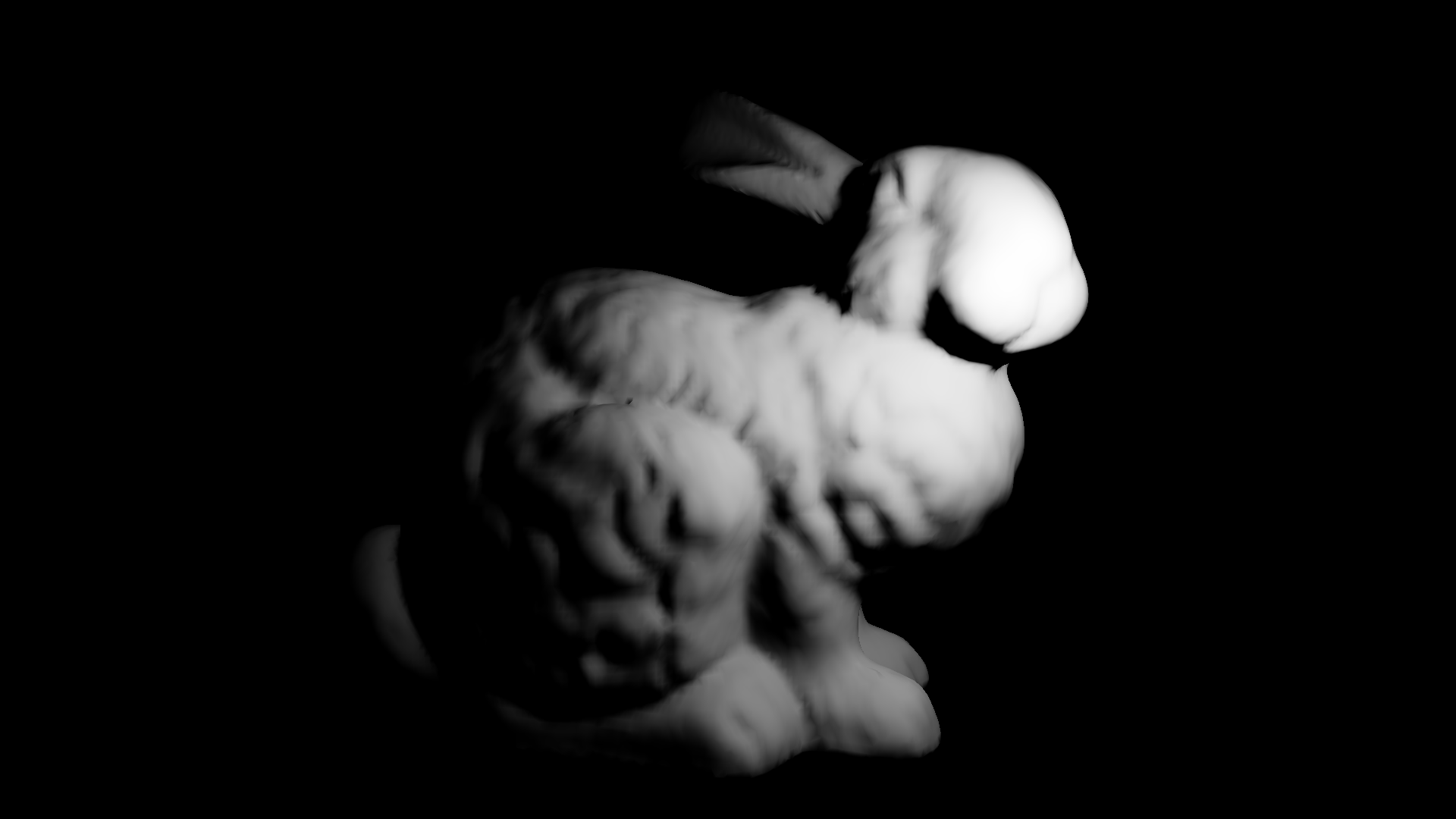## 彩色光源叠加 （左边黄色平行光，右边蓝色点光源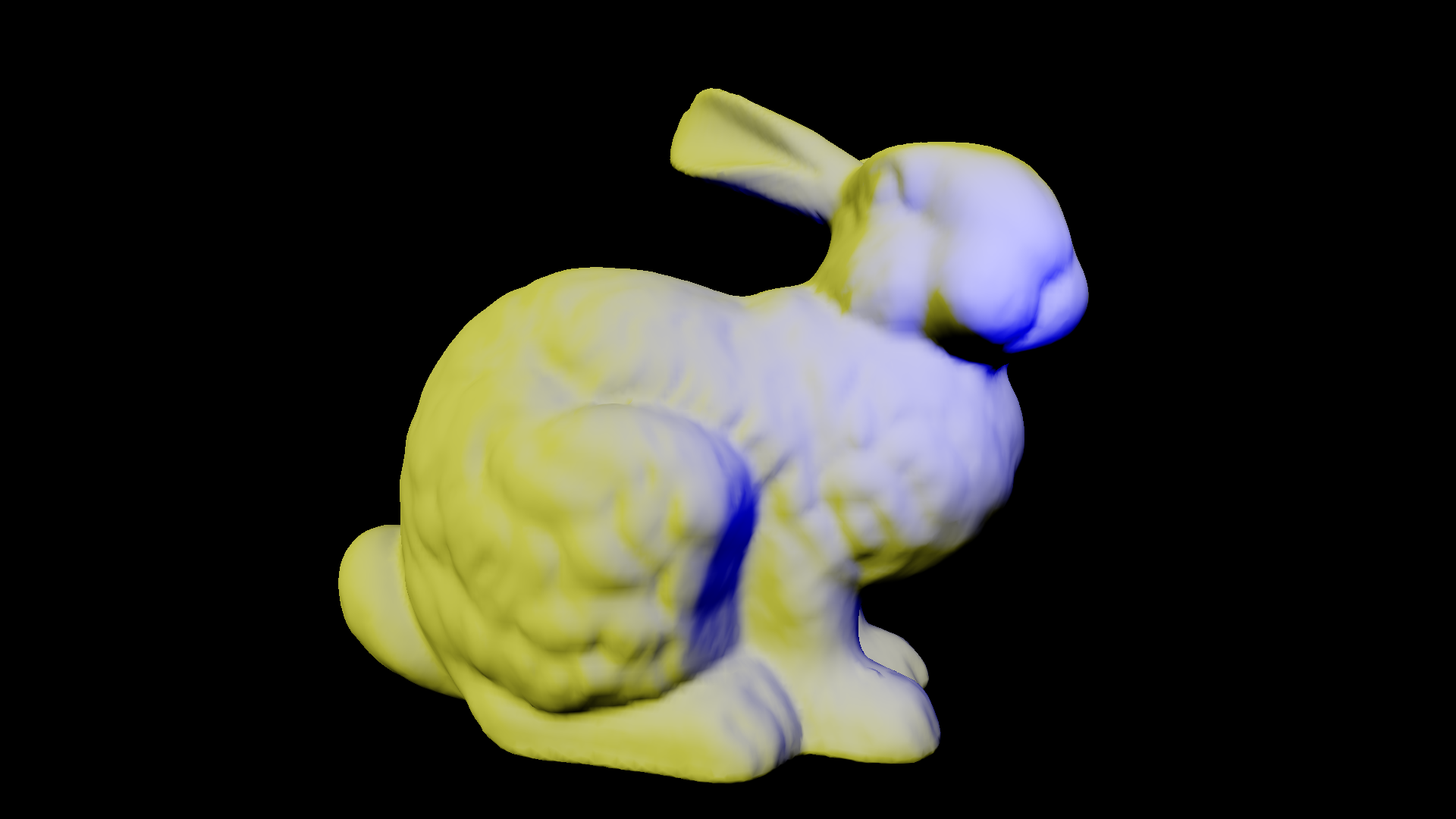# 配置文件格式

  1 2 3 4 5 6 7 8 9 10 11 12 13 14 15 16 17 18 19 20 21 22 23 24 25 26 27 28 29 30 31 32 33 34  { "width" : 1920, 宽 "height" : 1080, 高 "camera" :{ 相机参数 "position" : [0.800000, 0.800000, 0.800000], 位置 "u" : [0.707106, -0.707106, 0], 右向量 "v" : [-0.408248, -0.408248, 0.816496], 上向量 "n" : [-0.577349, -0.577349, -0.577349], 前向量 "fov" : 80, 视角 "near" : 0.1, 近裁剪面 "far" : 1000 远裁剪面 }, "object" :[ 模型参数 { "path" : "cube.obj", obj文件目录 "position" : [0.000000, 0.000000, 0.000000], 位置 "u" : [1, 0, 0], 同相机 "v" : [0, 1, 0], "n" : [0, 0, 1] } ], "light" :[ 光源参数 { "type" : "DirectLight", 方向光 "direction" : [-0.267261, -0.534522, -0.801784],方向 "color" : [1, 1, 1] 颜色 }, { "type" : "PointLight", 点光源 "position" : [1, 1, 1], 位置 "color" : [0.5, 0.5, 0.5] 颜色 } ] }

《3D游戏编程大师技巧》